# Themes, topics - math word problems

1. Two boats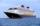The sightseeing boat sail at 25 km / h. The cruise take 6 hours. How long the another boat sails at 60 km/h?
2. PumpingThe tank is filled with a larger pump in 12 hours and with scond pump in 15 hours. For how long does the tank fill when both pumps are switched on at the same time?
3. Resultant forceCalculate mathematically and graphically the resultant of a three forces with a common centre if: F1 = 50 kN α1 = 30° F2 = 40 kN α2 = 45° F3 = 40 kN α3 = 25°
4. Diver and pressure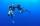Determine what pressures is exposed diver without a suit at a depth of 15 meters, with a small space suit at a depth of 25 meters and a massive suit at a depth of 70 m in water.
5. Forces on earth directionsA force of 60 N [North] and 80 N [East] is exerted on an object wigth 10 kg. What is the acceleration of the object?
6. King's birthday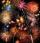To celebrate the king's birthday workers fire 1/5 all purchased rockets. To celebrate the Queen's birthday fire 1/6 of the remaining rockets and to celebrate the birthday of king's son remaining 15,000 rockets. How many rockets they purchased?
7. TruckThe truck passed 4550 km in 5 days. The first three days passed every day the same way. The fourth day passed 630 km and the fifth day was 920 km. How many km has passed the first three days?
8. DriverI drive at an average speed of 70km/h, I drive 10 thousand km. How long did it take me?
9. Working time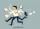At 10 hours work shift, work is done in 16 days. How many days will the work be done at 8 hours shifts?
10. CoolantThe driver of the car cooler filled with a mixture of 3.9 liters and 2.6 liters of water antifreeze coolant. At what rate are this two components of the mixture?
11. Trapezoidal prismCalculate the surface of the quadrilateral prism ABCDA'B'C'D 'with the trapezoidal base ABCD. The height of the prism is 12 cm; ABCD trapezoidal data: AB base length is 8 cm, CD base length is 3 cm, BC arm length is 4 cm, and AC diagonal length is 7 cm. L
12. MO Z8-I-1 2018Fero and David meet daily in the elevator. One morning they found that if they multiply their current age, they get 238. If they did the same after four years, this product would be 378. Determine the sum of the current ages of Fero and David.
13. Distance between two citiesCar went from A to B 4h. On the way back, the car was up 15km / h faster. The return trip took 48 minutes. Shorter than the way there. Find the distance of the cities A and B.
14. StormSo far, a storm has traveled 35 miles in 1/2 hour in direction straight to observer. If it is currently 5:00 p. M. And the storm is 105 miles away from you, at what time will the storm reach you? Explain how you solved the problem.
15. Three paintersThe three painters have painted bridge. The first would work done in 5 days, the second in 6 days, and the third in 7.5 days. How long will the bridge work if they work together?
16. MO Z8–I–6 2018In the KLMN trapeze, KL has a 40 cm base and an MN of 16 cm. Point P lies on the KL line so that the NP segment divides the trapezoid into two parts with the same area. Find the length of the KP line.
17. Wagons and cranesSeveral of the same cranes unloaded 96 wagons. If there were 2 more cranes there would be less 8 wagons for each crane. How many cranes were here?
18. Free fall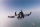How long does the stone fall freely into a depth of 80m? What speed will it hit the bottom of the abyss?
19. PumpsAfter the floods, four equally powerful pumps exhausted water from the flooded cellar in 6 hours. How many hours would take a drained out with three equally powerful pumps?
20. 3 cats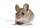3 cats eat 3 mice in 3 days. How many mice will eat 10 cats in 10 days?

Do you have an interesting mathematical word problem that you can't solve it? Enter it, and we can try to solve it.

To this e-mail address, we will reply solution; solved examples are also published here. Please enter the e-mail correctly and check whether you don't have a full mailbox.

Please do not submit problems from current active competitions such as Mathematical Olympiad, correspondence seminars etc...